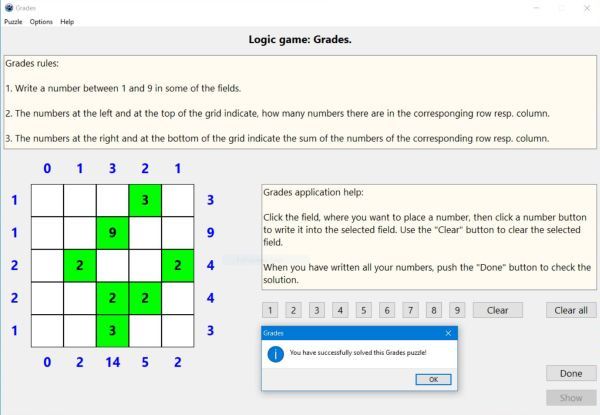# Computing: Free Pascal Programming

## Logic number puzzle: Grades.

Description: Puzzle game with numbers to be placed onto a grid. The rules of the game are as follows:
1. Write a number between 1 and 9 in some of the fields.
2. The numbers at the left and at the top of the grid indicate the numbers count per row resp. per column.
3. The numbers at the right and at the bottom of the grid indicate the numbers sum per row resp. per column.
The game is a typical logic game: In order to find the correct solution of the puzzle, you have to explore the count and sum values, and by logical thinking (concluding from what you know, that a given field must be a given number, or on the contrary, can't be a given number), determine, to which fields the numbers have to be written and what's their value.
The game is played with the mouse: Click the field, where you want to write a number, then click one of the 9 number buttons to write the corresponding number or Clear to remove the number from the actually selected field.

Note: The Grades application uses simplified game rules. In fact, in the original game, there is one more condition, not allowing that the number fields touch each other. Perhaps implementing this (as an option) in a future version...

Free Pascal features: Changing the color of shapes and the caption of static-texts during runtime. Using buttons to insert characters into a given control's caption. Two-dimensional arrays (classic Pascal).

Screenshot: